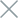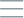• Home
• Tags
• Series
•# State-Based Search Algorithm in Java

The State-Based Search algorithm is a search method in Java programming that involves creating and traversing through possible states of a problem. In this algorithm, all possible states of a problem are represented as nodes in a graph or state space.

## How State-Based Search Algorithm Works

The algorithm starts from an initial state and uses transformations to generate child states. Each newly generated state becomes a node in the graph or state space. The algorithm traverses through these states, checking if the goal state is among them. If found, the algorithm terminates; otherwise, it continues traversing through other child states.

• Exhaustive: The algorithm has the capability to cover all possible states of the problem.
• Versatile: It can be applied to various types of problems.

• Possibility of repetition: In some cases, the algorithm might repeat the traversal of certain states.

## Example and Explanation

An illustrative example of the State-Based Search Algorithm is finding a path from a starting point to a destination on a map. Let's see how this algorithm works:

``````import java.util.*;

public class StateSearchExample {
static boolean isGoalState(State state, State goalState) {
return state.equals(goalState);
}

static void stateSearch(State initialState, State goalState) {
Set<State> visited = new HashSet<>();

while (!queue.isEmpty()) {
State currentState = queue.poll();

if (isGoalState(currentState, goalState)) {
System.out.println("Found goal state: " + currentState);
return;
}

List<State> nextStates = currentState.generateNextStates();
for (State nextState : nextStates) {
if (!visited.contains(nextState)) {